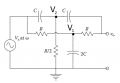# Parallel-T null circuit question - nodal analysis, finding the frequency

Joined Dec 21, 2015
67Find the frequency w0 at which V0 → 0.

Attempt at solution
(V2-Vs)jwC + 2V2/R + (V2-Vo)jwC = 0
(2V_2-Vs-Vo)jwC + 2V_2/R = 0 [eq 1]

2V_1-Vs-Vo+2RjwCV_1 = 0
[eq 2]

Combining both equations: jwC(2V_2-2RjwCV_1)+2V_2/R = 0
2V_2(jwC+1/R) + (wC)^2*2RV_1 = 0

Did I go wrong somewhere? Also, how are sub/superscripts done on this forum?
\$test = 1/(2\pi*L_{3}C_{c})\$

#### The Electrician

Joined Oct 9, 2007
2,914
Besides the ground reference node, you have 4 nodes: Vs, V1, V2, and Vo.

You will need 4 equations. After you have solved for the 4 node voltages, form an expression for the ratio Vo/Vs and calculate the frequency where that ratio becomes zero.

•#### MrAl

Joined Jun 17, 2014
9,623
View attachment 171034
Find the frequency w0 at which V0 → 0.

Attempt at solution
(V2-Vs)jwC + 2V2/R + (V2-Vo)jwC = 0
(2V_2-Vs-Vo)jwC + 2V_2/R = 0 [eq 1]

2V_1-Vs-Vo+2RjwCV_1 = 0
[eq 2]

Combining both equations: jwC(2V_2-2RjwCV_1)+2V_2/R = 0
2V_2(jwC+1/R) + (wC)^2*2RV_1 = 0

Did I go wrong somewhere? Also, how are sub/superscripts done on this forum?
\$test = 1/(2\pi*L_{3}C_{c})\$
Hi,

Yeah you need at least one more equation for Vo. That gives you three equations in three unknowns, then solve for Vo. You'll get three different values for w and perhaps only one of them is real.
If you substitute all the values for R and C with equivalents as shown in your schematic though you get a second order response which has two solutions for w and only one of them is positive and it comes out very simple.
So we have basically a third order response but once it is tuned perfectly (with all exact values for R and C and 2*C and R/2) we get a second order response.

Another issue that comes up though is what happens when we introduce some source resistance and some load resistance. The expression becomes more complicated and the sharpness and frequency symmetricalness depends on these values as well.

Last edited:
•